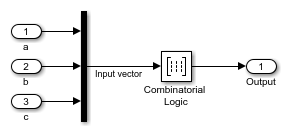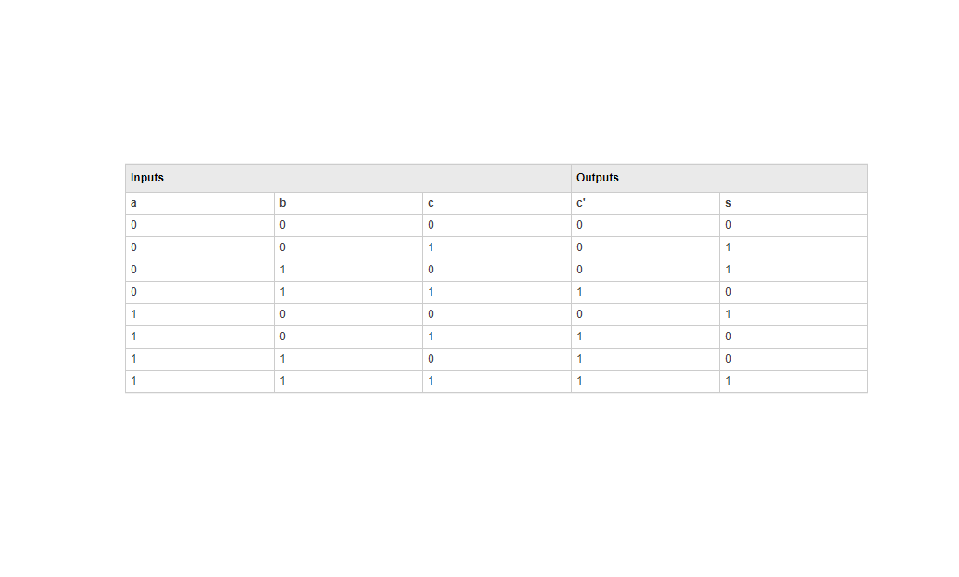Circuit Logic

This sample circuit has three inputs: the two bits ( a and b) to be summed and a carry-in bit (c). It has two outputs: the carry-out bit (c') and the sum bit (s).The truth table and corresponding outputs for each combination of input values for this circuit appear in the following table.To implement this adder with the Combinatorial Logic block, you enter the 8-by-2 matrix formed by columns c' and s as the Truth table parameter. You can also implement sequential circuits (that is, circuits with states) with the Combinatorial Logic block by including an additional input for the state of the block and feeding the output of the block back into this state input.